•5星
29KB m0_52957036 2020-05-09 20:12:07
•5星
11.8MB guoruibin123 2021-05-21 15:45:32
• 利用matlab实现非线性拟合（三维、高维、参数方程） matlab 非线性拟合 线性拟合

利用matlab实现非线性拟合0 前言1 线性拟合1.1 多项式拟合1.2 线性拟合2 一维非线性拟合2.1 简单的非线性拟合2.2 matlab中Curve Fitting App2.3 matlab中非线性拟合的实现2.3.1 fit()函数2.3.2 nlinfit()函数2.3.3 ...

2021.06 更新。补充了非线性拟合中，关于最小二乘定义的问题，放在了最后一章。
2021.12更新。应评论区建议，补充了3.3章绘图用的代码。

本文首发于 matlab爱好者 微信公众号，欢迎关注。

惯例声明：本人没有相关的工程应用经验，只是纯粹对相关算法感兴趣才写此博客。所以如果有错误，欢迎在评论区指正，不胜感激。本文主要关注于算法的实现，对于实际应用等问题本人没有任何经验，所以也不再涉及。

本人在学习matlab匿名函数时，作为练习有感而发，写下下面内容，非专业，难免有误。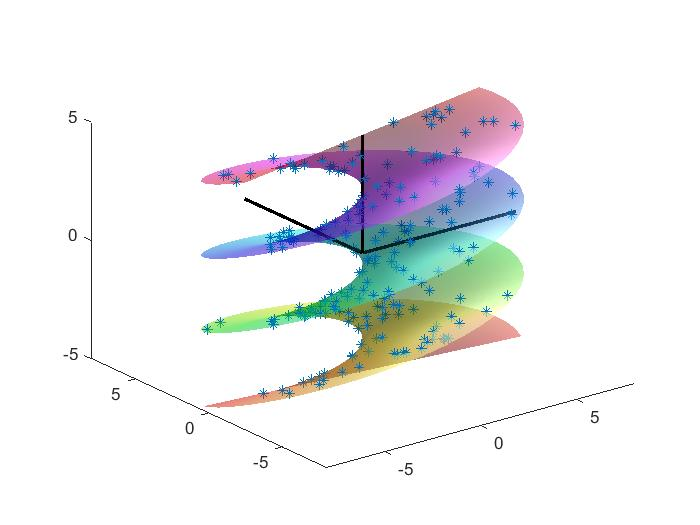0 前言

一般而言，通过已有的数据点去推导其它数据点，常见的方法有插值和拟合。插值适用性较广，尤其是线性插值或样条插值已被广泛的应用。但是通过已知的函数去拟合数据，是连接理论与实验重要的桥梁，这一点是插值无法替代的。

日常学习工作中，经常会遇到下面这种问题：想要用某个具体函数去拟合自己的数据，明明知道这个函数的具体形式，却不知道其中的参数怎么选取。本文就简单介绍一下matlab环境下，如何进行非线性拟合。

1 线性拟合

1.1 多项式拟合

多项式拟合就是利用下面形式的方程去拟合数据：
y = a + b x + c x 2 + d x 3 + . . . y=a +bx+cx^2+dx^3+...
matlab中可以用polyfit()函数进行多项式拟合。下面举一个小例子：

对于已有的数据点，我们采用4阶多项式拟合。其中已知函数的的表达式为

y=0.03 x^4 - 0.5 x^3 + 2 x^2 - 4

在此基础上添加了一些噪声点。拟合曲线依然采用4阶进行拟合，结果如下。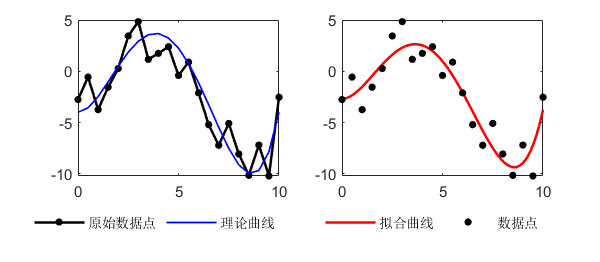可以看到拟合曲线与理论曲线基本一致，说明这种方法能够较好的拟合出原始数据的趋势。源代码如下：

x=0:0.5:10;
y=0.03*x.^4-0.5*x.^3+2.0*x.^2-0*x-4+6*(rand(size(x))-0.5);
p=polyfit(x,y,4);
x2=0:0.05:10;
y2=polyval(p,x2);
figure();
subplot(1,2,1)
hold on
plot(x,y,'linewidth',1.5,'MarkerSize',15,'Marker','.','color','k')
plot(x,0.03*x.^4-0.5*x.^3+2.0*x.^2-0*x-4,'linewidth',1,'color','b')
hold off
legend('原始数据点','理论曲线','Location','southoutside','Orientation','horizontal')
legend('boxoff')
box on
subplot(1,2,2)
hold on
plot(x2,y2,'-','linewidth',1.5,'color','r')
plot(x,y,'LineStyle','none','MarkerSize',15,'Marker','.','color','k')
hold off
box on
legend('拟合曲线','数据点','Location','southoutside','Orientation','horizontal')
legend('boxoff')

对于多项式拟合，不是阶数越多越好。比如还是这个上面这个例子，阶数越多，曲线越能够穿过每一个点，但是曲线的形状与理论曲线偏离越大。所以选择最适合的才是最好的。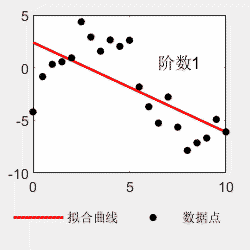1.2 线性拟合

线性拟合就是能够把拟合函数写成下面这种形式的：
y = p 1 f 1 ( x ) + p 2 f 2 ( x ) + p 3 f 3 ( x ) + . . . y=p_1 f_1(x) +p_2 f_2(x)+p_3 f_{3}(x)+...

其中f(x)是关于x的函数，其表达式是已知的。p是常数系数，这个是未知的。

对于这种形式的拟合，matlab内部有一个及其强悍的函数，可以自动输出p的解，并且满足最小二乘。这个函数就是\。没错，就是斜杠。这个符号通常用于求解方程AX=B的情况，我们用X=A\B可以求出未知数X。我们利用当A行和列不等时，输出X的最小二乘这个特性，就可以求出相应的最佳拟合。

还是举个例子虽然看上去很非线性，但是x和y都是已知的，a、b、c是未知的，而且是线性的，所以可以被非常简单的拟合出来。假设a=2.5，b=0.5，c=-1，加入随机扰动。拟合的最终效果为：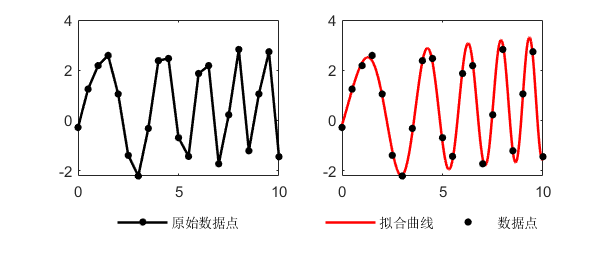最终得到的拟合参数为：a=2.47，b=0.47，c=-0.66。考虑到随机噪声的影响，与原始数据相差不大，源代码如下：

%线性拟合
x=0:0.5:10;
a=2.5;
b=0.5;
c=-1;
%原函数
y=a*sin(0.2*x.^2+x)+b*sqrt(x+1)+c+0.5*rand(size(x));

%拟合部分
yn1=sin(0.2*x.^2+x);
yn2=sqrt(x+1);
yn3=ones(size(x));
X=[yn1;yn2;yn3];
%拟合，得到系数
Pn=X'\y';
yn=Pn(1)*yn1+Pn(2)*yn2+Pn(3)*yn3;

%绘图
figure()
subplot(1,2,1)
plot(x,y,'linewidth',1.5,'MarkerSize',15,'Marker','.','color','k')
legend('原始数据点','Location','southoutside','Orientation','horizontal')
legend('boxoff')
subplot(1,2,2)
hold on
x2=0:0.01:10;
plot(x2,Pn(1)*sin(0.2*x2.^2+x2)+Pn(2)*sqrt(x2+1)+Pn(3),'-','linewidth',1.5,'color','r')
plot(x,y,'LineStyle','none','MarkerSize',15,'Marker','.','color','k')
hold off
box on
legend('拟合曲线','数据点','Location','southoutside','Orientation','horizontal')
legend('boxoff')

事实上，其实常用的拟合方式中，有很多都是线性拟合，比如多项式拟合，傅里叶拟合等。对于多项式拟合，只需要把拟合的部分替换成x，x.^2，x.^3这种形式。对于傅里叶级数，只需要把拟合的部分替换为sin(x)，sin(2*x)，sin(3*x)这种形式就行。

下面展示一个利用线性拟合，进行不同频率的三角波级数拟合正弦函数的例子：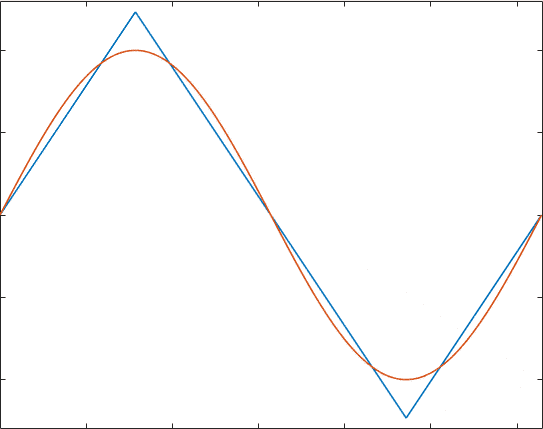clear;
clc;
close all
t=0:0.001:2*pi;%原函数
YS=sin(t);%基函数
N=21;
Yo=[];
for k=1:N
Yn=sawtooth(k*(t+pi/2),0.5);
Yo=[Yo,Yn'];
end
YS=YS';%拟合
a = Yo\YS;
%绘图
figure()
for k=1:N
clf
plot(t,Yo(:,1:k)*a(1:k,:),t,YS,'LineWidth',1)
ylim([-1.3,1.3])
xlim([0,6.3])
pause(0.1)
end

2 一维非线性拟合

2.1 简单的非线性拟合

前面介绍了线性的拟合方法。如果一个非线性方程，可以化为上面线性方程中公式中给出的样子，那么我们也可以套用线性的方法去求解。

比如下面的方程：
y = a ∗ exp ⁡ ( − b x ) y=a*\exp(-bx)
经过取对数变换，可以变为下面等效的线性形式：
lg ⁡ ( y ) = lg ⁡ ( a ) + b ∗ ( − x ) \lg(y)=\lg(a)+b*(-x)
这样求出来之后，再反变换回去，就可以得到原方程的系数了。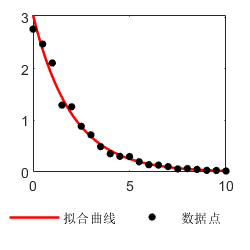代码如下：

clear
clc
close all

%方法1
x=0:0.5:10;
a=2.5;
b=0.5;
%原函数
y=a*exp(-b*x);
y=y+0.5*y.*rand(size(y));%加噪声
%变形函数
%Lg_y=Lg_a+b*(-x)
Lg_y=log(y);
%拟合部分
yn1=ones(size(x));
yn2=-x;
X=[yn1;yn2];
%拟合，得到系数
Pn=X'\Lg_y';
%反变换
a_fit=exp(Pn(1));
b_fit=Pn(2);
%绘图
figure()
hold on
x2=0:0.01:10;
plot(x2,a_fit*exp(-b_fit*x2),'-','linewidth',1.5,'color','r')
plot(x,y,'LineStyle','none','MarkerSize',15,'Marker','.','color','k')
hold off

2.2 matlab中Curve Fitting App

matlab自带了一个Curve Fitting App，它在matlab集成的App里面。界面左边输入数据，右侧选择方法。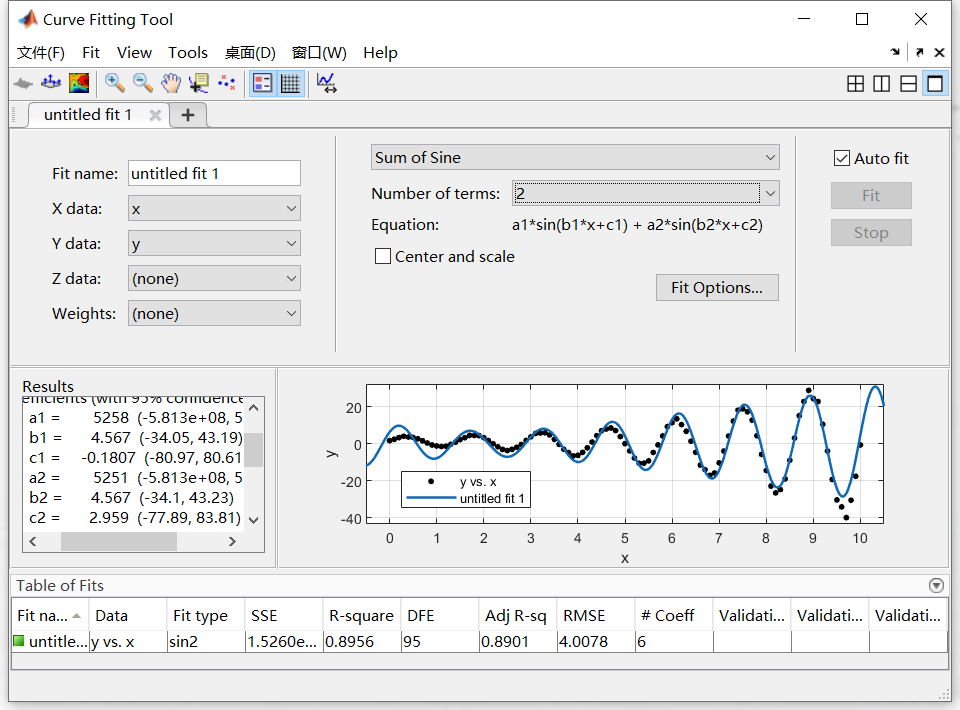界面里常用的拟合方式都有，而且直接展示拟合效果，非常方便。非常适合鼠标直接拖拖拽拽点点点的操作方式。

2.3 matlab中非线性拟合的实现

2.3.1 fit()函数

matlab中，fit()函数是一个比较通用的用于函数拟合的函数。它的优点就是非常的全面，可以用于各种种类的拟合。上面的App里，很多拟合种类都是间接调用了fit函数来实现的拟合。

对于非线性拟合，可以使用fit()函数中的Nonlinear Least Squares方法。其大概原理为，首先确定一个初始的点，计算该点的雅可比矩阵J，来近似线性化的判断该点周围的趋势，并将这个点向更小的方向移动。

因此，这个方法的一个缺点在于，对于初始点的选取非常敏感，最终结果只能在初始点附近的局部最小值点上，而不能保证全局最小值。

2.3.2 nlinfit()函数

相比于前面的fit()函数，nlinfit()函数是matlab专门的非线性拟合函数。对于非稳健估计，采用的是Levenberg-Marquardt（LM）方法，也叫阻尼最小二乘法。对于稳健估计，采用的是Iteratively Reweighted Least Squares方法，也就是在Least Squares基础上，对每一个拟合点的权重进行调整的一种方法。这两者方法也都是基于雅克比矩阵的方法。

2.3.3 lsqnonlin()函数和lsqcurvefit()函数

lsqnonlin()也是matlab中自带的一个非线性拟合函数。它给出了两种计算非线性拟合的方法，一种是比较经典之前介绍过的LM方法，一种是信赖域方法。信赖域法（trust region reflective）是通过Hessian 矩阵，逐步试探邻域内的最小化，来求解问题的。相比较之前的那些雅克比相关的方法，信赖域法会占用更多内存和速度，所以适用于中小规模的矩阵。

lsqcurvefit()函数和lsqnonlin()内容上相似，只是引用格式上有所不同。

2.3.4 fsolve()函数

这也是一个求解非线性方程的函数，可以求解方程组或者矩阵形式，功能非常强大。默认的算法为trust-region-dogleg，俗称狗腿法，属于信赖域法。这里用到的功能比较基础，所以也不过多介绍了。

2.3.5 粒子群算法

说了那么多，发现逐渐从如何非线性拟合，陷入到了最优化的深坑里。而且前面的那么多方法，很多都解决不了陷入到局部最优解的问题。实际上，这种问题如果进入了最优化领域，很多智能算法也可以被考虑进来。所以我也把粒子群PSO算法加入到了里面，尝试将结果收敛到全局最优解。

2.3.6 不同算法的对比效果

第一个例子是 y=a.*exp(-b\*(x-c).^2)+d，一个简单的高斯函数形式的非线性方程，其参数给定为：

abcd
3.82.14.4-1.3

在已知函数形式，求解这四个参数条件下，6种不同的函数非拟合效果如下：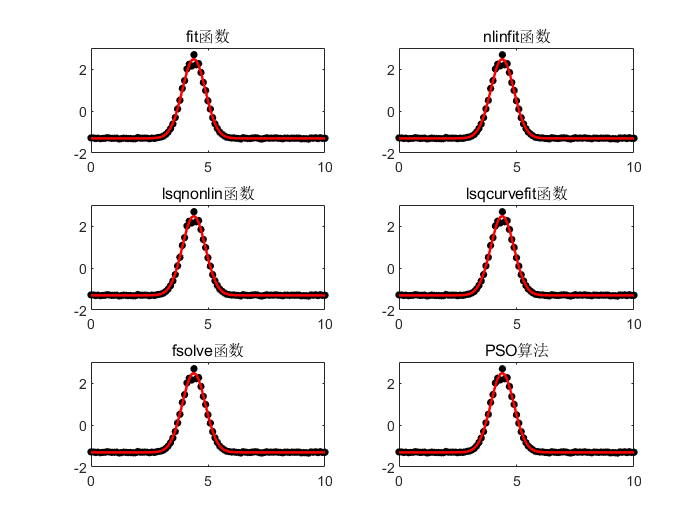可以看到，这几种方法都能够较好的拟合出想要的结果。

第二个例子是一个指数增长的正弦函数，在很多线性系统中都可以测量到这种信号。函数的形式为：

y=a*x+b*sin(c*x).*exp(d*x)+e 。其给定的参数为：

abcde
-0.32.14.40.31.7

这个函数的拟合具有一定难度，拟合过程中会遇到非常多的局部解。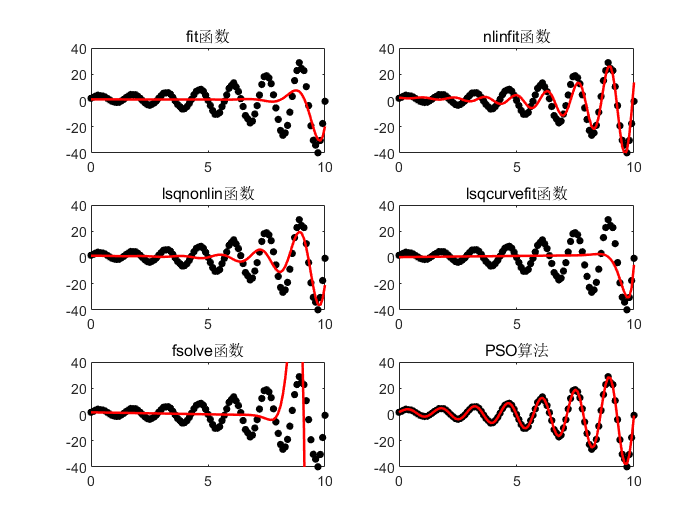由于初始点比较随机，所以最终结果每次都会不一样。
其中前面的几种方法对于初始值的敏感度比较高，如果初始值选的比较接近原始解，也是可以得到较好的结果。其中nlinfit函数经常会报错，容错率较低。而PSO算法经常能够收敛到最优解（虽然不是每次都可以，偶尔也会陷入局部解）。

代码如下：

clear
clc
%函数大比拼
close all

%初始设置
x = 0:0.1:10;
a = -0.3;
b = 2.1;
c = 4.4;
d = 0.3;
e = 1.7;

y = a*x+b*sin(c*x).*exp(d*x)+e;
noise = 0.05*abs(y-1).*randn(size(x));
y = y+noise;%加噪声函数

figure();%plot(x,y)
y_lim = [-40,40];

%% 1 fit()函数 Least Squares
ft = fittype( 'a*x+b*sin(c*x).*exp(d*x)+e', 'independent', 'x', 'dependent', 'y' );
OP1 = fitoptions( 'Method', 'NonlinearLeastSquares' );
OP1.StartPoint = 5*rand(1,5);%初始值，越靠近真实值越好
OP1.Lower = [-2,0,2,0,0];%参数的最小边界
OP1.Upper = [1,3,5,3,3];%参数的最大边界
%拟合
fitobject = fit(x',y',ft,OP1);
a2=fitobject.a;
b2=fitobject.b;
c2=fitobject.c;
d2=fitobject.d;
e2=fitobject.e;
%展示结果
y1 = a2*x+b2*sin(c2*x).*exp(d2*x)+e2;
subplot(3,2,1)
hold on
plot(x,y,'LineStyle','none','MarkerSize',15,'Marker','.','color','k')
plot(x,y1,'-','linewidth',1.5,'color','r')
hold off
box on
ylim(y_lim)
title('fit函数')

%% 2 nlinfit()函数 Levenberg-Marquardt %容易报错
modelfun = @(p,x)( p(1)*x+p(2)*sin(p(3)*x).*exp(p(4)*x)+p(5) );

OP2 = statset('nlinfit');
%opts.RobustWgtFun = 'bisquare';
p0 = 5*rand(1,5);
p = nlinfit(x,y,modelfun,p0,OP2);
%拟合
y2 = p(1)*x+p(2)*sin(p(3)*x).*exp(p(4)*x)+p(5);
subplot(3,2,2)
hold on
plot(x,y,'LineStyle','none','MarkerSize',15,'Marker','.','color','k')
plot(x,y2,'-','linewidth',1.5,'color','r')
hold off
box on
ylim(y_lim)
title('nlinfit函数')

%% 3 lsqnonlin()函数 trust-region-reflective
modelfun = @(p)(p(1)*x+p(2)*sin(p(3)*x).*exp(p(4)*x)+p(5) -y) ;%这里和nlinfit()函数定义不一样
p0 = 5*rand(1,5);
OP3 = optimoptions(@lsqnonlin,'Algorithm','trust-region-reflective','MaxFunctionEvaluations',1e4,'MaxIterations',1e3);
[p,~] = lsqnonlin(modelfun,p0,[-2,0,2,0,0],[1,3,5,3,3],OP3);
y3 = p(1)*x+p(2)*sin(p(3)*x).*exp(p(4)*x)+p(5);
subplot(3,2,3)
hold on
plot(x,y,'LineStyle','none','MarkerSize',15,'Marker','.','color','k')
plot(x,y3,'-','linewidth',1.5,'color','r')
hold off
box on
ylim(y_lim)
title('lsqnonlin函数')

%% 4 lsqcurvefit()函数 trust-region-reflective
modelfun = @(p,x)(p(1)*x+p(2)*sin(p(3)*x).*exp(p(4)*x)+p(5)) ;%这里和其它函数的定义也不一样
p0 = 5*rand(1,5);
OP4 = optimoptions('lsqcurvefit','Algorithm','trust-region-reflective','MaxFunctionEvaluations',1e4,'MaxIterations',1e3);
[p,~] = lsqcurvefit(modelfun,p0,x,y,[-2,0,2,0,0],[1,3,5,3,3],OP4);
y4 = p(1)*x+p(2)*sin(p(3)*x).*exp(p(4)*x)+p(5);
subplot(3,2,4)
hold on
plot(x,y,'LineStyle','none','MarkerSize',15,'Marker','.','color','k')
plot(x,y4,'-','linewidth',1.5,'color','r')
hold off
box on
ylim(y_lim)
title('lsqcurvefit函数')

%% 5 fsolve()函数 %默认算法trust-region-dogleg
modelfun = @(p)(p(1)*x+p(2)*sin(p(3)*x).*exp(p(4)*x)+p(5) -y);
p0 = 5*rand(1,5);
OP5 = optimoptions('fsolve','Display','off');
p = fsolve(modelfun,p0,OP5);
y5 = p(1)*x+p(2)*sin(p(3)*x).*exp(p(4)*x)+p(5);
subplot(3,2,5)
hold on
plot(x,y,'LineStyle','none','MarkerSize',15,'Marker','.','color','k')
plot(x,y5,'-','linewidth',1.5,'color','r')
hold off
box on
ylim(y_lim)
title('fsolve函数')

%% 6 粒子群PSO算法
fun = @(p) ( norm(p(1)*x+p(2)*sin(p(3)*x).*exp(p(4)*x)+p(5) -y) );%这里需要计算误差的平方和
OP6 = optimoptions('particleswarm','InertiaRange',[0.4,1.2],'SwarmSize',100);
[p,~,~,~]  = particleswarm(fun,5,[-5,-5,-5,-5],[5,5,5,5],OP6);%区间可以稍微放大一些，不怕
y6 = p(1)*x+p(2)*sin(p(3)*x).*exp(p(4)*x)+p(5);
subplot(3,2,6)
hold on
plot(x,y,'LineStyle','none','MarkerSize',15,'Marker','.','color','k')
plot(x,y6,'-','linewidth',1.5,'color','r')
hold off
box on
ylim(y_lim)
title('PSO算法')

下图展示了PSO求解过程中逐渐收敛到全局最优解的过程。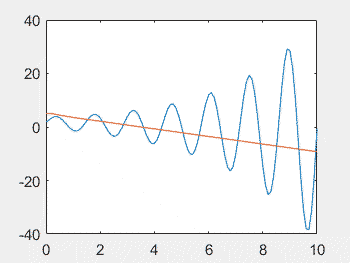3 高维非线性方程组拟合

3.1 一般形式高维方程或方程组的拟合

之前的文章中的数据具有一 一对应的特点，所以严格来讲并不是普遍的二维拟合。对于一些复杂的二维函数，比如椭圆，可能原本的拟合就会失效。

对于这种一般性质的方程或方程组，将原本输入方程整理为f(x,y,z,…)=0的形式。衡量拟合程度的优化函数，就直接取函数f(xi,yi,zi,…)的值即可。

下面演示最终的两个例子：

第一个是三维直线，采用两平面式描述。

Ax+By+Cz-1=0
Dx+Ey+Fz-1=0

总共2个方程，维度为3维，第一个方程有3个参数，第二个方程也有3个参数。离散点已知的条件下，三维直线的两平面表达式不唯一。

最终拟合效果如下：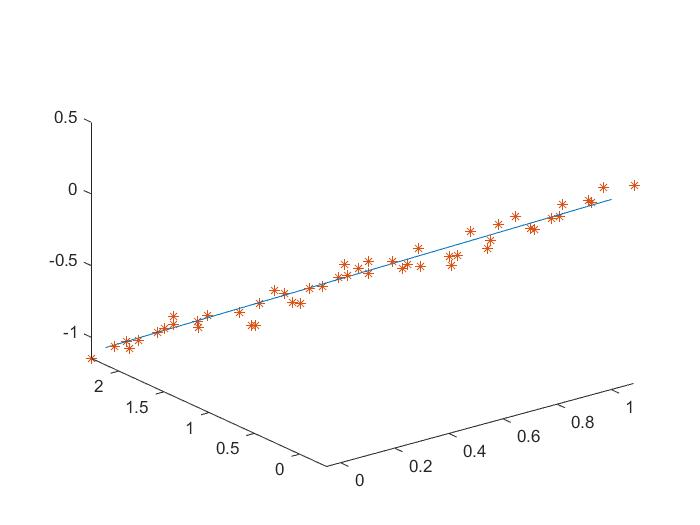第二个是二维椭圆，方程为：

x^2+Axy+By^2+Cx+Dy+E=0

总共1个方程，维度为2维。方程共有5个参数。

最终拟合效果如下：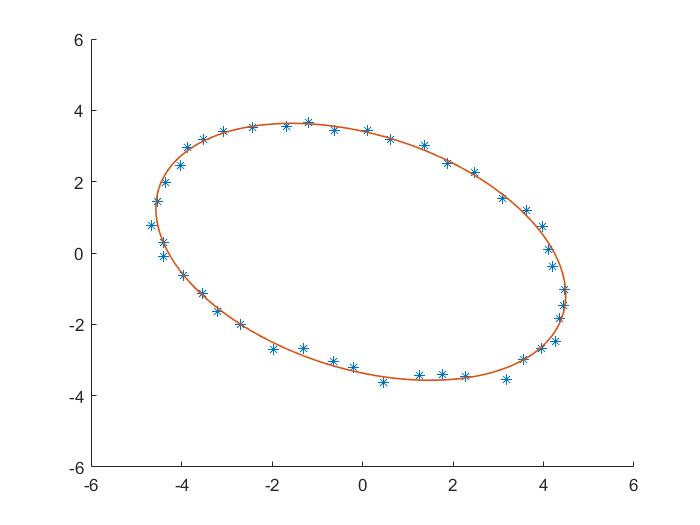clear
clc
close all
%% 演示1
%1 导入数据（这里用的是人工生成的数据）
%三维直线拟合，函数表示
%1.0*x+1.9*y+3.0*z=1;
%1.2*x-0.4*y-1.7*z=1;
x=0:0.04:1;
%求解出y和z
% [ 1.0, 3.0    [ y        [1.0
%  -0.4,-1.7] *   z] = 1 -  1.2] x
y=zeros(size(x));z=y;
for k=1:length(x)
R=[1.9,3.0;-0.4,-1.7]\[1-1.0*x(k);1-1.2*x(k)];
[y(k),z(k)]=Value(R);
end
rand_n=randperm(length(x));
%生成随机的初始输入数据
x1=x(rand_n)+0.05*randn(size(x));
y1=y(rand_n)+0.05*randn(size(x));
z1=z(rand_n)+0.05*randn(size(x));
%2 整理格式，将数据和要拟合的函数进行格式整理
%准备数据
XX={x1,y1,z1};
%准备函数
F1=@(p1,XX1) p1(1)*XX1{1}+p1(2)*XX1{2}+p1(3)*XX1{3}-1;
F2=@(p2,XX2) p2(1)*XX2{1}+p2(2)*XX2{2}+p2(3)*XX2{3}-1;
FF={F1,F2};
%3 生成最终优化函数，带入到优化方程中求解
fun=@(p) Dis(p,{3,3},XX,FF);%p参数，{3,3}第一个方程3个参数，第二个方程3个参数。XX离散点。FF函数表达式。
OP=optimoptions('particleswarm','FunctionTolerance',0);
[p,fval,~,~]=particleswarm(fun,6,[-5,-5,-5,-5,-5,-5],[5,5,5,5,5,5],OP);
%4 对比拟合效果
figure()
x2=0:0.01:1;
y2=zeros(size(x2));
z2=y2;
for k=1:length(x2)
R=[p(2),p(3);p(5),p(6)]\[1-p(1)*x2(k);1-p(4)*x2(k)];
[y2(k),z2(k)]=Value(R);
end
%系数可能不同。因为直线的两平面表示不唯一
hold on
plot3(x2,y2,z2)
plot3(x1,y1,z1,'*');
hold off
view(3)

%% 演示2
%1 导入数据（这里用的是人工生成的数据）
%二维椭圆拟合
th=0:0.15:2*pi;
a=3.2;%椭圆轴1
b=4.8;%椭圆轴2
x0=-1.9;
y0=-4.1;
beta=1.1;%椭圆旋转角度
%绘制椭圆
x=a*cos(th);
y=b*sin(th);
%旋转beta角度
x_r=x*cos(beta)-y*sin(beta);
y_r=x*sin(beta)+y*cos(beta);
rand_n=randperm(length(x));
%生成随机的初始输入数据
x1=x_r(rand_n)+0.1*randn(size(x));
y1=y_r(rand_n)+0.1*randn(size(x));
%2 整理格式，将数据和要拟合的函数进行格式整理
%准备数据
XX={x1,y1};
%准备函数
F1=@(p1,XX1) XX1{1}.*XX1{1}+p1(1)*XX1{1}.*XX1{2}+p1(2)*XX1{2}.*XX1{2}+p1(3)*XX1{1}+p1(4)*XX1{2}+p1(5);
FF={F1};
%3 生成最终优化函数，带入到优化方程中求解
fun=@(p) Dis(p,{5},XX,FF);
OP=optimoptions('particleswarm','FunctionTolerance',0);
[p,fval,~,~]=particleswarm(fun,5,[-20,-20,-20,-20,-20],[20,20,20,20,20],OP);
%4 对比拟合效果
figure()
hold on
plot(x1,y1,'*')
Fun_Plot=@(xp,yp) xp.*xp+p(1)*xp.*yp+p(2)*yp.*yp+p(3)*xp+p(4)*yp+p(5);
fimplicit(Fun_Plot,[-6 6 -6 6],'LineWidth',1)
hold off

%% 用到的函数
function varargout=Value(f)
%多元素赋值，例子：
%[a,b,c]=Value([1,2,3]);%多变量赋值
%[xy(1),xy(2),t]=Value([2,5,3]);%复合赋值
%[b,a]=Value([a,b]);%元素交换
%来源：hyhhhyh21，matlab爱好者
N=numel(f);
varargout=cell(1,N);
if nargout~=N
error('输入输出数量不一致')
end
for k=1:N
varargout{k}=f(k);
end
end

function Sum_N=Dis(p,p_num,XX,FF)
%用函数值评价拟合程度
%输入：要拟合的参数p
%输入：p_num cell格式，每个方程的参数数量
%输入：XX 数据，以cell形式储存
%输入：FF 拟合函数，以cell形式储存
N_F=numel(FF);%要联立的方程数量
L=length(XX{1});%离散点的数量
N_L=numel(XX);%离散点维度
Sum_N=0;
XXm=cell(1,N_L);
%直接计算函数的值
p_n=1;%参数的索引
for k=1:N_F
%对每一个方程进行计算
FF_k=FF{k};%方程
F_p=p_num{k};%该方程用到的参数数量
for m=1:L
for n=1:N_L
XXm{n}=XX{n}(m);
end
Distance=FF_k(p(p_n:(p_n+F_p-1)),XXm);
Sum_N=Sum_N+Distance^2;
end
p_n=p_n+F_p;%更新函数索引
end
end

3.2 一般形式参数方程的拟合

高维参数方程的拟合比较困难。因为原本方程中x、y、z的坐标点都是已知的。但是参数方程中，x、y、z的坐标点已知，但是与参数u、v往往未知。所以相当于原本的方程中引入了额外的未知信息。

但是基本思路和普通方程是一样的。离散点距离假想方程的距离，需要用该点距方程上每个点的距离中的最小值，来近似判断。

依然是展示两个例子。

第一个是计算三维李萨如图形。参数方程为：

x=sin(A*u)
y=cos(B*u)
z=sin(C*u)

方程为三维参数方程，只有一个参数u。第一个方程有1个未知量A，第二个方程有1个未知量B，第三方程有1个未知量C。

最终拟合效果如下。由于李萨如图形中，只要频率的比例固定，图案就会固定。所以最终ABC的值不唯一，但是它们的比例肯定唯一。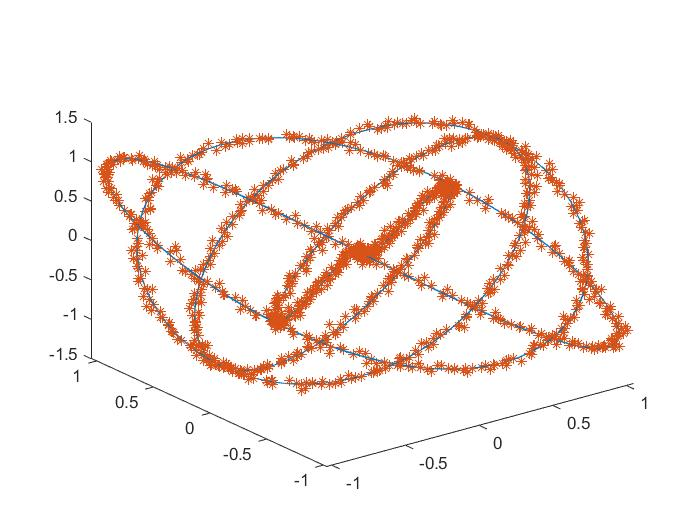第二个例子是一个三维旋转曲面。参数方程为：

x= A*u.*sin(v+B)
y=-C*u.*cos(v+D)
z=v

方程为三维参数方程，有2个参数u、v。第一个方程有2个未知量AB，第二个方程有2个未知量CD，第三方程有0个未知量。

最终拟合效果如下：这两个例子的演示代码如下。这里参数方程的Dis_P()由于频繁的计算点与点之间的距离，所以运算速度比第一章单纯函数的Dis()较慢。

clear
clc
close all
%% 演示1
%三维李萨如图形拟合
%1 导入数据（这里用的是人工生成的数据）
t=0:0.01:4*pi;
x=sin(4*t);
y=cos(5*t);
z=sin(6*t);

rand_n=randperm(length(t));
x1=x(rand_n)+0.02*randn(size(t));
y1=y(rand_n)+0.02*randn(size(t));
z1=z(rand_n)+0.02*randn(size(t));
%2 整理格式，将数据和要拟合的函数进行格式整理
%输入参数方程
XX={x1,y1,z1};%离散点输入
F1=@(p1,uu1) sin(p1(1)*uu1{1});
F2=@(p2,uu1) cos(p2(1)*uu1{1});
F3=@(p3,uu1) sin(p3(1)*uu1{1});
FF={F1,F2,F3};%方程输入
u1=0:0.05:13;%设置参数的最大最小范围以及精度，能达到绘图精度即可
uu={u1};%参数输入
%3 生成最终优化函数，带入到优化方程中求解
fun=@(p) Dis_P(p,{1,1,1},uu,XX,FF);%使得DisP函数输出的Sum_N最小
%p参数，{1,1,1}代表有3个方程，每个方程的代求参数p个数为1。uu为参数输入。XX为离散点输入。FF为方程输入。
OP=optimoptions('particleswarm','FunctionTolerance',0,'InertiaRange',[0.3,1.2],'MaxStallIterations',200);
[pp,fval,~,~]=particleswarm(fun,3,[1,1,1],[10,10,10],OP);
%4 对比拟合效果
figure()
hold on
tt=0:0.01:4*pi;
%pp=pp/pp(1)*4;%这里不一定必须是4,5,6，只需要比例为4:5:6就行
[a2,b2,c2]=Value(pp);
x2=sin(a2*tt);
y2=cos(b2*tt);
z2=sin(c2*tt);

plot3(x2,y2,z2);
plot3(x1,y1,z1,'*');
hold off
view(3)

%% 演示2
%三维螺旋面拟合
%1 导入数据（这里用的是人工生成的数据）
F1=@(p1,uu1) p1(1).*uu1{1}.*sin(uu1{2}+p1(2));
F2=@(p2,uu1) -p2(1).*uu1{1}.*cos(uu1{2}+p2(2));
F3=@(p3,uu1) uu1{2};

u_rand=rand_AB([-4,4],100,1);
v_rand=rand_AB([-5,5],100,1);
x=F1([2,0.1],{u_rand,v_rand});
y=F2([1,0.1],{u_rand,v_rand});
z=F3([],{u_rand,v_rand});

rand_n=randperm(length(x));
x1=x(rand_n)+0.01*randn(size(x));
y1=y(rand_n)+0.01*randn(size(x));
z1=z(rand_n)+0.01*randn(size(x));

%2 整理格式，将数据和要拟合的函数进行格式整理
%输入参数方程
XX={x1,y1,z1};%离散点输入
FF={F1,F2,F3};%方程输入
u1=-4:0.1:4;%设置参数的最大最小范围以及精度，能达到绘图精度即可
v1=-5:0.1:5;%设置参数的最大最小范围以及精度，能达到绘图精度即可
uu={u1,v1};%参数输入
%3 生成最终优化函数，带入到优化方程中求解
fun=@(p) Dis_P(p,{2,2,0},uu,XX,FF);%使得DisP函数输出的Sum_N最小
OP=optimoptions('particleswarm','FunctionTolerance',0,'InertiaRange',[0.3,1.2],'MaxStallIterations',200);
[pp,fval,~,~]=particleswarm(fun,4,[0.1,0,0.1,0],[10,2*pi,10,2*pi],OP);

%4 对比拟合效果
figure()
hold on
plot3(x1,y1,z1,'*');
funx = @(u,v) pp(1)*u.*sin(v+pp(2));
funy = @(u,v) -pp(3)*u.*cos(v+pp(4));
funz = @(u,v) v;
fsurf(funx,funy,funz,[-4 4 -5 5],'LineStyle','none','FaceAlpha',0.5)
xlim([-8,8]);
ylim([-8,8]);
zlim([-5,5]);
colormap(hsv)
camlight
plot3([0,8],[0,0],[0,0],'linewidth',2,'color','k')
plot3([0,0],[0,8],[0,0],'linewidth',2,'color','k')
plot3([0,0],[0,0],[0,5],'linewidth',2,'color','k')
hold off
view(3)

function Sum_N=Dis_P(p,p_num,uu,XX,FF)
%用距离曲线的距离评价拟合程度（参数方程）
%输入：p 要拟合的参数
%输入：p_num 数组，每个方程的参数数量
%输入：uu 参数方程中的参数，以cell形式储存
%输入：XX 数据，以cell形式储存
%输入：FF 拟合函数，以cell形式储存

N_F=numel(FF);%要联立的方程数量
L=length(XX{1});%离散点的数量
N_L=numel(XX);%拟合参数p的数量
N_u=numel(uu);%参数方程中参数的数量
if N_u>1
uu_new=ndgrid_h(uu{:});
uu=uu_new;
end
%循环每个点，求到直线的距离
%在假定参数p的情况下，计算假定函数
Point_NF=cell(N_F,1);
p_n=1;%参数的索引
for k=1:N_F
F_p=p_num{k};%该方程用到的参数数量
Point_NF{k}=FF{k}(p(p_n:(p_n+F_p-1)),uu);%计算假定函数各个点坐标
p_n=p_n+F_p;%更新函数索引
end
Sum_N=0;
for k=1:L
%分别求每个假定函数的点，与真实离散点之间距离的平方和
Point_distance2=0;
for m=1:N_F
%读取真实点坐标
Point_distance2=Point_distance2+(Point_NF{m}-XX{m}(k)).^2;
end
Min_distance2=min(Point_distance2);%求出最小距离，即为点与假定函数之间的距离
Sum_N=Sum_N+Min_distance2;
end
end

function varargout=Value(f)
%多元素赋值，例子：
%[a,b,c]=Value([1,2,3]);%多变量赋值
%[xy(1),xy(2),t]=Value([2,5,3]);%复合赋值
%[b,a]=Value([a,b]);%元素交换
%来源：hyhhhyh21，matlab爱好者
N=numel(f);
varargout=cell(1,N);
if nargout~=N
error('输入输出数量不一致')
end
for k=1:N
varargout{k}=f(k);
end
end

function X=rand_AB(AB,varargin)
%生成区间[A,B]之间的随机分布
%除了AB之外，其余输入与rand相同
[A,B]=Value(AB);
X=rand(varargin{1:end});
X=A+X*(B-A);
end

function S=ndgrid_h(varargin)
%来源于matlab自带的源代码。
%用法和ndgrid用法一样，但是将输出更改为cell
N=nargin;
S=cell(1,N);
if N==1
S{1}=varargin;
else
j = 1:N;
siz = cellfun(@numel,varargin);
if N == 2 % Optimized Case for 2 dimensions
x = full(varargin{1}(:));
y = full(varargin{2}(:)).';
S{1} = repmat(x,size(y));
S{2} = repmat(y,size(x));
else
for i=1:N
x = full(varargin{j(i)});
s = ones(1,N);
s(i) = numel(x);
x = reshape(x,s);
s = siz;
s(i) = 1;
S{i} = repmat(x,s);
end
end
end
S2=cell(1,N);
for k=1:N
S2{k}=S{k}(:);
end
S=S2;
end

主要函数就是Dis和Dis_P，准备工作就是把方程和离散点整理成可以输入的形式。优化用到的函数就是PSO（particleswarm），需要更改未知参数数量和范围就可以。

3.3 补充

这一部分的拟合优度或者置信区间就没办法拿出来说了，毕竟有试凑的嫌疑。适合单纯的验证。

另外这里和最小二乘求出来的结果会有所不同。这主要是定义的问题。其中最小二乘指的是距离y方向上最小的二乘距离。而这一章节中定义的最小二乘，是指的点到拟合曲线距离的最小二乘（有点类似于主成分分析）。虽然一般情况下两者并不会有太大差别，但是如果有误差的话，还请注意这一点，

如下图，这里是一个椭圆分布的离散点。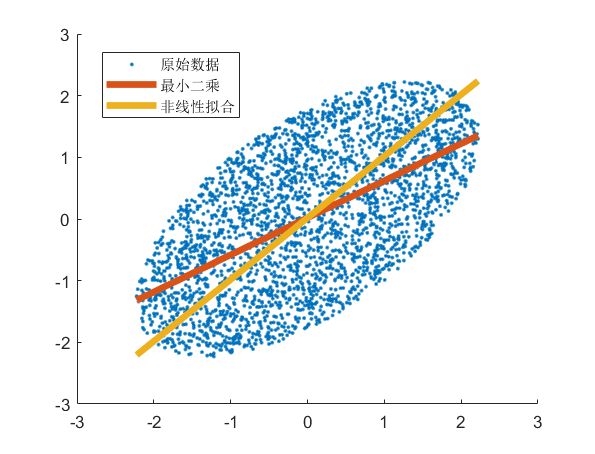如果将方程的形式定义为y=ax+b，要求距离y最小，则最终结果为红色的线。如果将方程定义为ax+by+c=0，要求距离直线的距离最小，则最终拟合结果为黄色的线。这里无所谓对错，只是要求的最小定义不同。

单独在后面补充了这么一个章节，希望在应用中注意。

上面的图，绘图代码如下：

%构建原始数据
t1=rand(3000,1);t2=rand(3000,1);
x=2*sqrt(t2).*cos(2*pi*t1);
y=sqrt(t2).*sin(2*pi*t1);
XY=[x,y];
XY2=XY*[1,1;-1,1];%变形
x2=XY2(:,1);y2=XY2(:,2);
x=x2;y=y2;
%% 演示1
%线性最小二乘拟合，以|y-yi|^2作为衡量指标
yn1=x;
yn2=ones(size(x));
X=[yn1,yn2];
%拟合，得到系数
Pn=X\y;
yn=Pn(1)*yn1+Pn(2)*yn2;
%% 演示2
%二维直线非线性拟合，以|ax+by+c|^2作为衡量指标
%准备数据
XX={x,y};
%准备函数
F1=@(p1,XX1) p1(1)*XX1{1}-p1(2)*XX1{2}+p1(3);%ax-by+c=0
FF={F1};
%3 生成最终优化函数，带入到优化方程中求解
fun=@(p) Dis(p,{3},XX,FF);%p参数，{2}第一个方程2个参数。XX离散点。FF函数表达式。
% Sum_N=Dis([1,0],{2},XX,FF)
OP=optimoptions('particleswarm','FunctionTolerance',0);
[p,fval,~,~]=particleswarm(fun,3,[1,1,-1],[5,5,1],OP);
%% 对比
figure()
hold on
plot(x,y,'.')
plot(x,yn,'linewidth',4)
plot(x,(p(1)*x+p(3))/p(2),'linewidth',4)
hold off
legend({'原始数据','最小二乘','非线性拟合'})

参考文献

展开全文weixin_42943114 2021-05-14 19:41:29
• 怎么用Python将图像边界用最小二乘法拟合成曲线本文实例讲述了Python基于最小二乘法实现曲线拟合。分享给大家供大家参考，具体如下： 这里不手动实现最小二乘，调用scipy库中实现好的相关优化函数。 考虑如下的含有4...

怎么用Python将图像边界用最小二乘法拟合成曲线

本文实例讲述了Python基于最小二乘法实现曲线拟合。分享给大家供大家参考，具体如下： 这里不手动实现最小二乘，调用scipy库中实现好的相关优化函数。 考虑如下的含有4个参数的函数式： 构造数据 ? 123456789101112131415 import numpy as npfro

如何用Python进行线性回归以及误差分析

数据挖掘中的预测问题通常分为2类：回归与分类。 简单的说回归就是预测数值，而分类是给数据打上标签归类。 本文讲述如何用Python进行基本的数据拟合，以及如何对拟合结果的误差进行分析。 本例中使用一个2次函数加上随机的扰动来生成500个点。

python中用polyfit拟合出的函数怎么能直接调用？

我用z1 = np.polyfit(x, y, 9)拟合出一个多项式，之后想直接用i=sympy.s首先分两种情况： 1.交互窗口处执行：这个时候由于python的强制缩进，因此想要结束函数的定义只需要按两下enter即可。 2.在.py文件中编写，结束函数只需要不再缩进即可 调用函数方法相同，把函数名及参数写上就可以了。

python怎样做高斯拟合

Python怎么实现非线性的拟合

import matplotlib.pyplot as ptimport numpy as npfrom scipy.optimize import leastsqfrom pylab import *time = []counts = []for i in open('/some/folder/to/file.txt', 'r'): segs = i.split() time.append(float(segs)) counts.append

Python 怎么用曲线拟合数据

我有两组数据： y = [41.417, 49.077, 26.683, 42.137, 37.31, 10.022, Python中利用guiqwt进行曲线数据拟合。

python怎么用线性回归拟合

python如何CSS布局HTML小编今天和大家分享一条拟合曲线的曲率最大点

拟合了这样的一条曲线，如何CSS布局HTML小编今天和大家分享这台曲线曲率的最大值，在图上标出最大点通过所给数据点信息得到的各组数据拟合曲线曲率最大值和位置如下 数据组别拟合点数拟合点范围最大曲率曲率最大值点(x) 第一组80.04~0.208.034150.088013 第二组90.04~0.406.3893060.11067 第三组110.04~0.804.3585720.162234 第四组100.05~0.8

python可以做数据拟合吗

准确的来说是做统计数据的可视化，一般的数据可视化都是js生成的，这点跟后端语言没啥关系。R的最大优点就是有一些优秀的可视化包。

展开全文weixin_32073865 2021-02-03 04:42:53
• 展开全部matlab中线性拟合可以使来用多项自式拟合函数polyfit()实现bai，du其调用格式为P=polyfit(X,Y,N)其中zhi，X，Y是需要拟合的数据，N是拟dao合的多项式的最高次数，P是拟合的多项式的系数。实例演示如下：1、...

展开全部

matlab中线性拟合可以使来用多项自式拟合函数polyfit()实现bai，du其调用格式为P = polyfit(X,Y,N)

其中zhi，X，Y是需要拟合的数据，N是拟dao合的多项式的最高次数，P是拟合的多项式的系数。

实例演示如下：

1、示例数据

x=[0.1,0.2,0.3,0.4,0.5,0.6,0.7,0.8,0.9,1.0,1.1,1.2]

y=[-8,-236,-415,-562,-701,-860,-961,-1082,-1188,-1304,-1405,-1534]

拟合方程：y=a+bx

2、matlab代码>> x=[0.1,0.2,0.3,0.4,0.5,0.6,0.7,0.8,0.9,1.0,1.1,1.2];

>> y=[-8,-236,-415,-562,-701,-860,-961,-1082,-1188,-1304,-1405,-1534];

>> p = polyfit(x,y,1)

>> plot(x,y,'o',x,polyval(p,x))

3、结果及说明p =

1.0e+003 *

-1.3253    0.0068

表示拟合的线性公式为 ： y = -1325.3x+6.8展开全文weixin_39960319 2020-12-24 03:59:08
• 使用“regress” 线性的不行，用二次函数。 format long A=[... 0.2 13.6 8503 251 27.4 7.7 9.9 3658 314 13.9 5.8 10.8 7307 433 26.8 7.70 9.70 6717 257 23.8 7.5 9.8 7609 280 21.7 ...

辛苦几天收集的资料：

1.使用“nlinfit”

x1=1150,1000,900,850,700,625,550,475,3350,3500,5900,5800,5700,4600,4625,4725,11650,11200,11200]';

x2=[175,100,25,0,75,100,150,200,50,600,500,225,100,1225,1600,2000,1200,1000,1550

]';

x=[x1,x2];

y=[1.44E-02,1.80E-02,6.08E-02,5.59E-02,3.42E-02,7.74E-03,1.17E-03,6.16E-03,1.91E-04,1.91E-04,1.02E-03,2.83E-03,9.52E-05,3.77E-04,2.70E-04,1.87E-04,3.98E-04,4.04E-04,4.02E-04]';

beta0=[0.1 0.1 1 1];

myfun=@(a,x)4030.0./pi./4.2./(a(1).*x(:,1).^a(2).*a(3).*x(:,1).^a(4)).*exp(-(x(:,2).^2./2./(a(1).*x(:,1).^a(2)).^2+30.0.^2./2./(a(3).*x(:,1).^a(4)).^2));

[a,b,c,d,res]=nlinfit(x,y,myfun,beta0);a,res

plot3(x1,x2,y,'o',x1,x2,myfun(a,x))

值的选取没有定法，与实际问题的模型有关。

2.使用“regress”

线性的不行，用二次函数。

format long

A=[...

0.2 13.6 8503 251 27.4 7.7 9.9 3658 314 13.9 5.8 10.8 7307 433 26.8 7.70 9.70 6717 257 23.8 7.5 9.8 7609 280 21.7 5.6 11.3 4271 533 14.6 6.2 7.6 52169 48 225 3.23 9.16 16516 80 44.1 0.33 11.3 17366 85 54.1 0.14 9.5 14245 91 56.6 5.5 9.7 18184 3 31.6 2.3 8.9 33612 250 114.9 3.3 4.6 73927 5 166 1.9 9.7 32175 150 107.5 0.6 9.9 33088 242 142.3 0.22 11.7 18620 567 60.4 1.88 11.76 27885 267 71.6 2.78 10.9 21780 76 58.7]

x=A(:,1:4),Y=A(:,5)

x11=x(:,1).*x(:,1);

x12=x(:,1).*x(:,2);

x13=x(:,1).*x(:,3);

x14=x(:,1).*x(:,4);

x22=x(:,2).*x(:,2);

x23=x(:,2).*x(:,3);

x24=x(:,2).*x(:,4);

x33=x(:,3).*x(:,3);

x34=x(:,3).*x(:,4);

x44=x(:,4).*x(:,4);

X=[x(:,:),x11,x12,x13,x14,x22,x23,x24,x33,x34,x44]

[B,BINT,R] = REGRESS(Y,[ones(length(Y),1),X]) 结果：

B =

1.0e+003 *

-1.426098928217992

-0.004076772421011

0.255534919787513

0.000012942581436

0.000845938681439

0.000607150442496

-0.000574488595437

0.000000405451807

-0.000042626483419

-0.011775830339062

-0.000000876232149

0.000008150156703

-0.000000000013441

-0.000000013991054

-0.000000969496753

R =

3.122573422039807

0.447341267999400

-7.343326306615449

2.107836742251767

-6.239492394117182

9.044235126157025

2.238791755625499

4.285551199892858

-2.231536057549363

-1.979307925154075

3.503835830046878

1.414933242530537

-1.426757776398972

-12.052007973319576

14.597045597468522

-5.024612350970848

-1.747668123505179

-2.717435276394376

B就是系数，R就是预测值与实际值的差值。

3. 使用“lsqcurvefit”

clear

clc

x=[40 50 60 70 80 90 100 110 120 135 150];

y=[0.0096 0.0145 0.0194 0.0348 0.0501 0.0751 0.1000 0.1497 0.1993 0.2496 0.2999];

z=[0.2400 0.2865 0.3330 0.3600 0.3870 0.4010 0.4150 0.4390 0.4630 0.4875 0.5120];

X0=[1 1 1 1 1 1];

%只要这样写就可以了

f=@(p,x)( p(1) + p(2)*x(1,:) +

p(3)*x(2,:) + p(4)*x(1,:).^2 + p(5)*x(1,:).*x(2,:) +

p(6)*x(2,:).^2);

p=lsqcurvefit(f,X0,[x;y],z)

展开全文weixin_34417272 2021-04-20 10:48:21
• weixin_33657090 2021-04-26 11:42:18
• weixin_39526651 2020-12-22 17:38:10
• [MATLAB 在科学计算中的应用] 使用MATLAB 进行非线性拟合 拟合工具箱 MATLAB

lusongno1 2020-12-22 01:07:39
• weixin_42395669 2021-04-20 10:48:20
• python 线性相关 与 线性拟合 python

Forrest97 2021-06-26 21:13:05
• qq_34159047 2021-05-15 15:09:34
• Python实现数据的线性拟合 线性代数 算法 python

LiDarF 2021-06-21 20:06:27
• weixin_30505255 2021-03-17 14:50:57
• 最小二乘法一阶线性拟合二阶曲线拟合的C语言程序实现.pdf 线性拟合的c语言程序

weixin_30015509 2021-05-19 19:57:50
• weixin_39531780 2021-04-18 04:56:57
• weixin_42521276 2021-04-20 04:13:34
• weixin_39959298 2021-01-14 05:26:24
• weixin_42126749 2021-04-19 04:51:48
• ziixiaoshenwang 2021-04-20 22:08:25
• weixin_34410975 2021-02-04 02:24:06
• weixin_39842271 2021-04-18 12:01:33
• weixin_39612817 2020-12-20 13:35:39
• weixin_32831131 2021-03-18 15:32:55
• Libtorch之神经网络线性拟合（C++/Qt） 深度学习 c++ 线性拟合 Qt Libtorch

qq_31793023 2021-11-23 21:41:57
• 对现有的股票数据进行线性拟合并用matplotlib绘图 python 机器学习 大数据 人工智能 数据分析

weixin_45081640 2021-06-07 17:56:12
• weixin_35900818 2021-04-20 14:14:26
• weixin_30932183 2021-04-20 15:30:41
• 数值计算之 拟合法，线性拟合，多项式拟合 线性代数 矩阵 机器学习

qq_41035283 2021-11-22 11:42:28
• 线性拟合过程动态图 python 机器学习 可视化

shuai_yue 2020-12-22 08:12:19
• PHP 二元线性拟合函数 php excel LINEST 线性拟合

csdn_Info 2021-07-31 12:37:47
• 最小二乘法之一元线性拟合 java 直线拟合

weixin_34013327 2021-02-26 10:11:38...